Data Structures
Topics:
Basics of Hash Tables
• Arrays
• Stacks
• Queues
• Hash Tables
• Basics of Hash Tables
• Trees
• Disjoint Data Structures

# Basics of Hash Tables

Hashing is a technique that is used to uniquely identify a specific object from a group of similar objects. Some examples of how hashing is used in our lives include:

• In universities, each student is assigned a unique roll number that can be used to retrieve information about them.
• In libraries, each book is assigned a unique number that can be used to determine information about the book, such as its exact position in the library or the users it has been issued to etc.

In both these examples the students and books were hashed to a unique number.

Assume that you have an object and you want to assign a key to it to make searching easy. To store the key/value pair, you can use a simple array like a data structure where keys (integers) can be used directly as an index to store values. However, in cases where the keys are large and cannot be used directly as an index, you should use hashing.

In hashing, large keys are converted into small keys by using hash functions. The values are then stored in a data structure called hash table. The idea of hashing is to distribute entries (key/value pairs) uniformly across an array. Each element is assigned a key (converted key). By using that key you can access the element in O(1) time. Using the key, the algorithm (hash function) computes an index that suggests where an entry can be found or inserted.

Hashing is implemented in two steps:

1. An element is converted into an integer by using a hash function. This element can be used as an index to store the original element, which falls into the hash table.
2. The element is stored in the hash table where it can be quickly retrieved using hashed key.

hash = hashfunc(key)
index = hash % array_size

In this method, the hash is independent of the array size and it is then reduced to an index (a number between 0 and array_size − 1) by using the modulo operator (%).

Hash function
A hash function is any function that can be used to map a data set of an arbitrary size to a data set of a fixed size, which falls into the hash table. The values returned by a hash function are called hash values, hash codes, hash sums, or simply hashes.

To achieve a good hashing mechanism, It is important to have a good hash function with the following basic requirements:

1. Easy to compute: It should be easy to compute and must not become an algorithm in itself.

2. Uniform distribution: It should provide a uniform distribution across the hash table and should not result in clustering.

3. Less collisions: Collisions occur when pairs of elements are mapped to the same hash value. These should be avoided.

Note: Irrespective of how good a hash function is, collisions are bound to occur. Therefore, to maintain the performance of a hash table, it is important to manage collisions through various collision resolution techniques.

Need for a good hash function

Let us understand the need for a good hash function. Assume that you have to store strings in the hash table by using the hashing technique {“abcdef”, “bcdefa”, “cdefab” , “defabc” }.

To compute the index for storing the strings, use a hash function that states the following:

The index for a specific string will be equal to the sum of the ASCII values of the characters modulo 599.

As 599 is a prime number, it will reduce the possibility of indexing different strings (collisions). It is recommended that you use prime numbers in case of modulo. The ASCII values of a, b, c, d, e, and f are 97, 98, 99, 100, 101, and 102 respectively. Since all the strings contain the same characters with different permutations, the sum will 599.

The hash function will compute the same index for all the strings and the strings will be stored in the hash table in the following format. As the index of all the strings is the same, you can create a list on that index and insert all the strings in that list.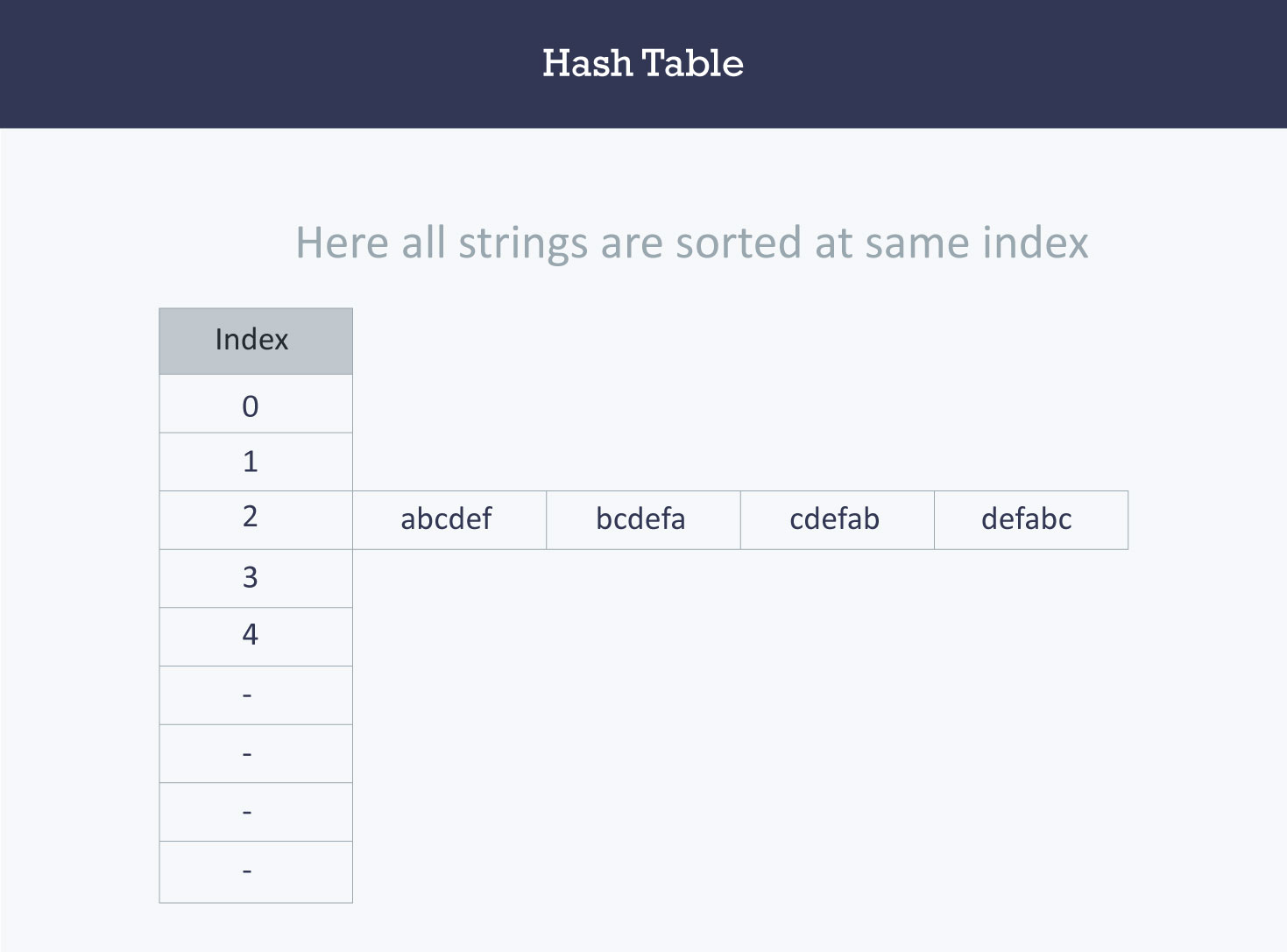Here, it will take O(n) time (where n is the number of strings) to access a specific string. This shows that the hash function is not a good hash function.

Let’s try a different hash function. The index for a specific string will be equal to sum of ASCII values of characters multiplied by their respective order in the string after which it is modulo with 2069 (prime number).

String                                Hash function                               Index
abcdef       (971 + 982 + 993 + 1004 + 1015 + 1026)%2069       38
bcdefa       (981 + 992 + 1003 + 1014 + 1025 + 976)%2069       23
cdefab       (991 + 1002 + 1013 + 1024 + 975 + 986)%2069       14
defabc       (1001 + 1012 + 1023 + 974 + 985 + 996)%2069       11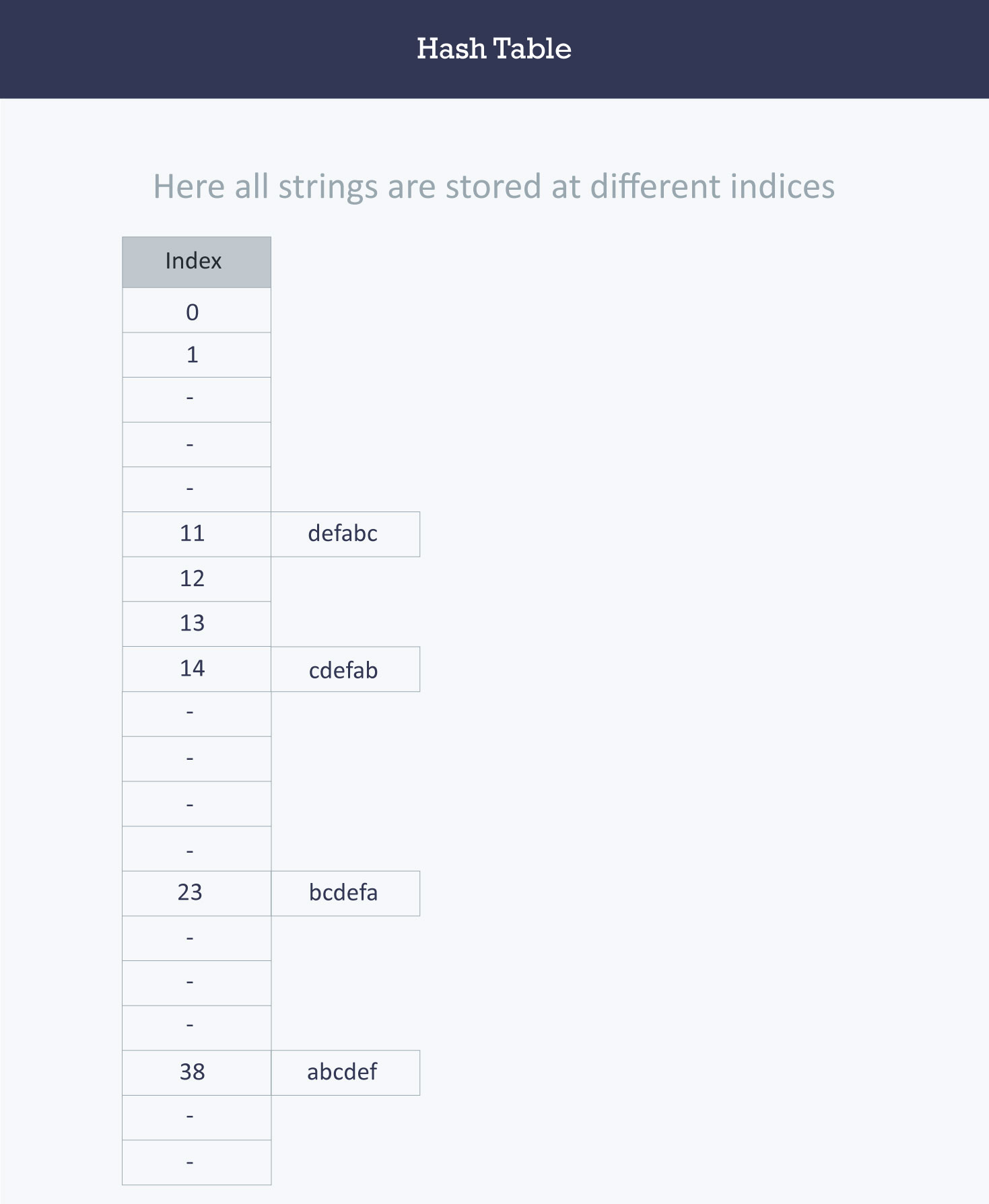Hash table
A hash table is a data structure that is used to store keys/value pairs. It uses a hash function to compute an index into an array in which an element will be inserted or searched. By using a good hash function, hashing can work well. Under reasonable assumptions, the average time required to search for an element in a hash table is O(1).

Let us consider string S. You are required to count the frequency of all the characters in this string.

string S = “ababcd”


The simplest way to do this is to iterate over all the possible characters and count their frequency one by one. The time complexity of this approach is O(26*N) where N is the size of the string and there are 26 possible characters.

  void countFre(string S)
{
for(char c = ‘a’;c <= ‘z’;++c)
{
int frequency = 0;
for(int i = 0;i < S.length();++i)
if(S[i] == c)
frequency++;
cout << c << ‘ ‘ << frequency << endl;
}
}


Output

a 2
b 2
c 1
d 1
e 0
f 0
…
z 0


Let us apply hashing to this problem. Take an array frequency of size 26 and hash the 26 characters with indices of the array by using the hash function. Then, iterate over the string and increase the value in the frequency at the corresponding index for each character. The complexity of this approach is O(N) where N is the size of the string.

 int Frequency;

int hashFunc(char c)
{
return (c - ‘a’);
}

void countFre(string S)
{
for(int i = 0;i < S.length();++i)
{
int index = hashFunc(S[i]);
Frequency[index]++;
}
for(int i = 0;i < 26;++i)
cout << (char)(i+’a’) << ‘ ‘ << Frequency[i] << endl;
}


Output

a 2
b 2
c 1
d 1
e 0
f 0
…
z 0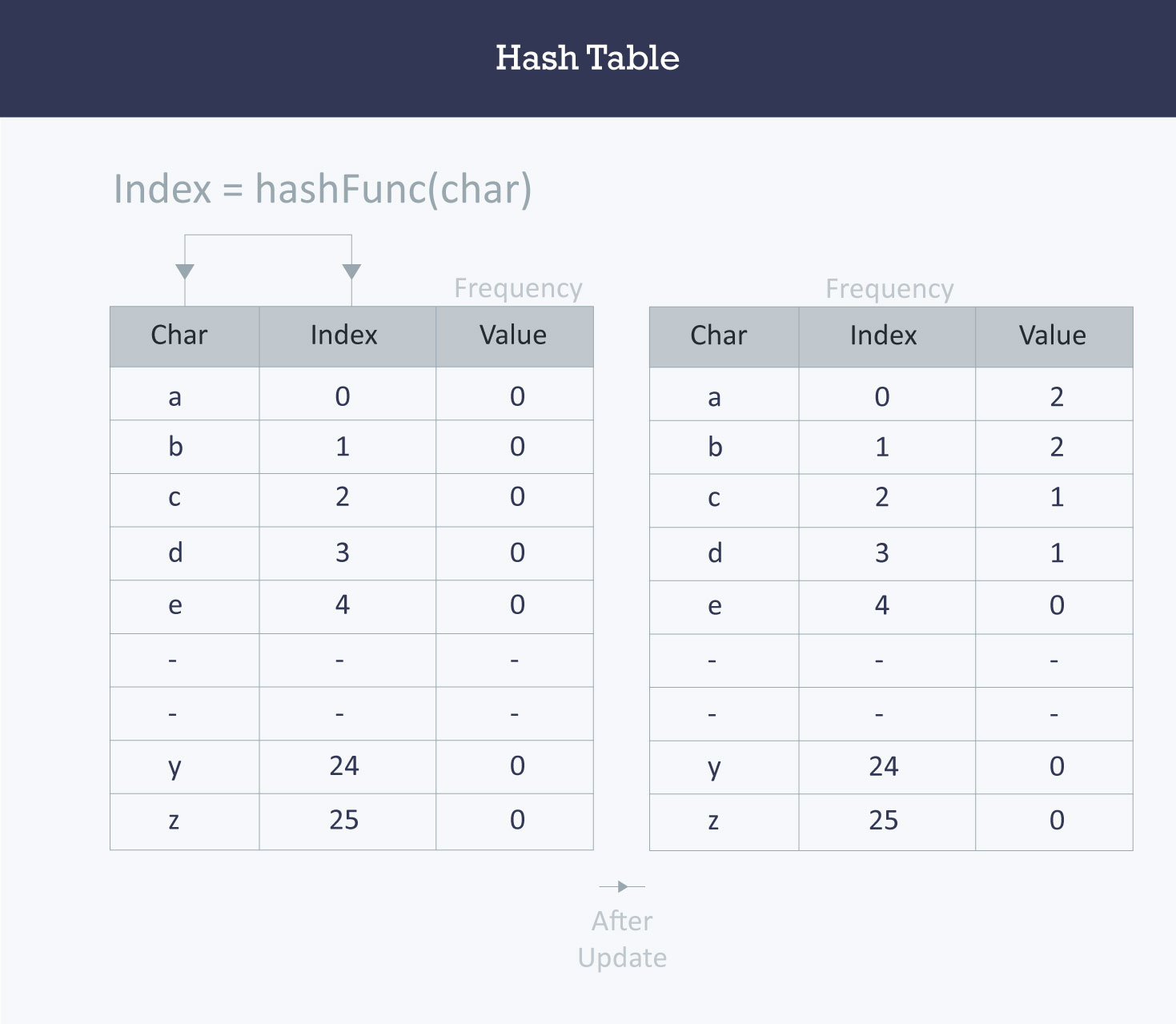Collision resolution techniques

Separate chaining (open hashing)

Separate chaining is one of the most commonly used collision resolution techniques. It is usually implemented using linked lists. In separate chaining, each element of the hash table is a linked list. To store an element in the hash table you must insert it into a specific linked list. If there is any collision (i.e. two different elements have same hash value) then store both the elements in the same linked list.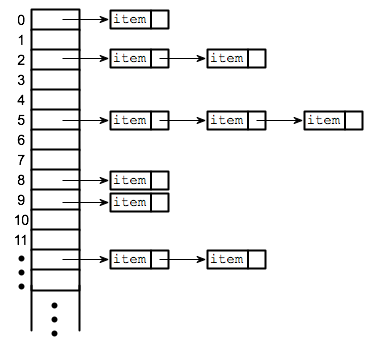The cost of a lookup is that of scanning the entries of the selected linked list for the required key. If the distribution of the keys is sufficiently uniform, then the average cost of a lookup depends only on the average number of keys per linked list. For this reason, chained hash tables remain effective even when the number of table entries (N) is much higher than the number of slots.

For separate chaining, the worst-case scenario is when all the entries are inserted into the same linked list. The lookup procedure may have to scan all its entries, so the worst-case cost is proportional to the number (N) of entries in the table.

In the following image, CodeMonk and Hashing both hash to the value 2. The linked list at the index 2 can hold only one entry, therefore, the next entry (in this case Hashing) is linked (attached) to the entry of CodeMonk.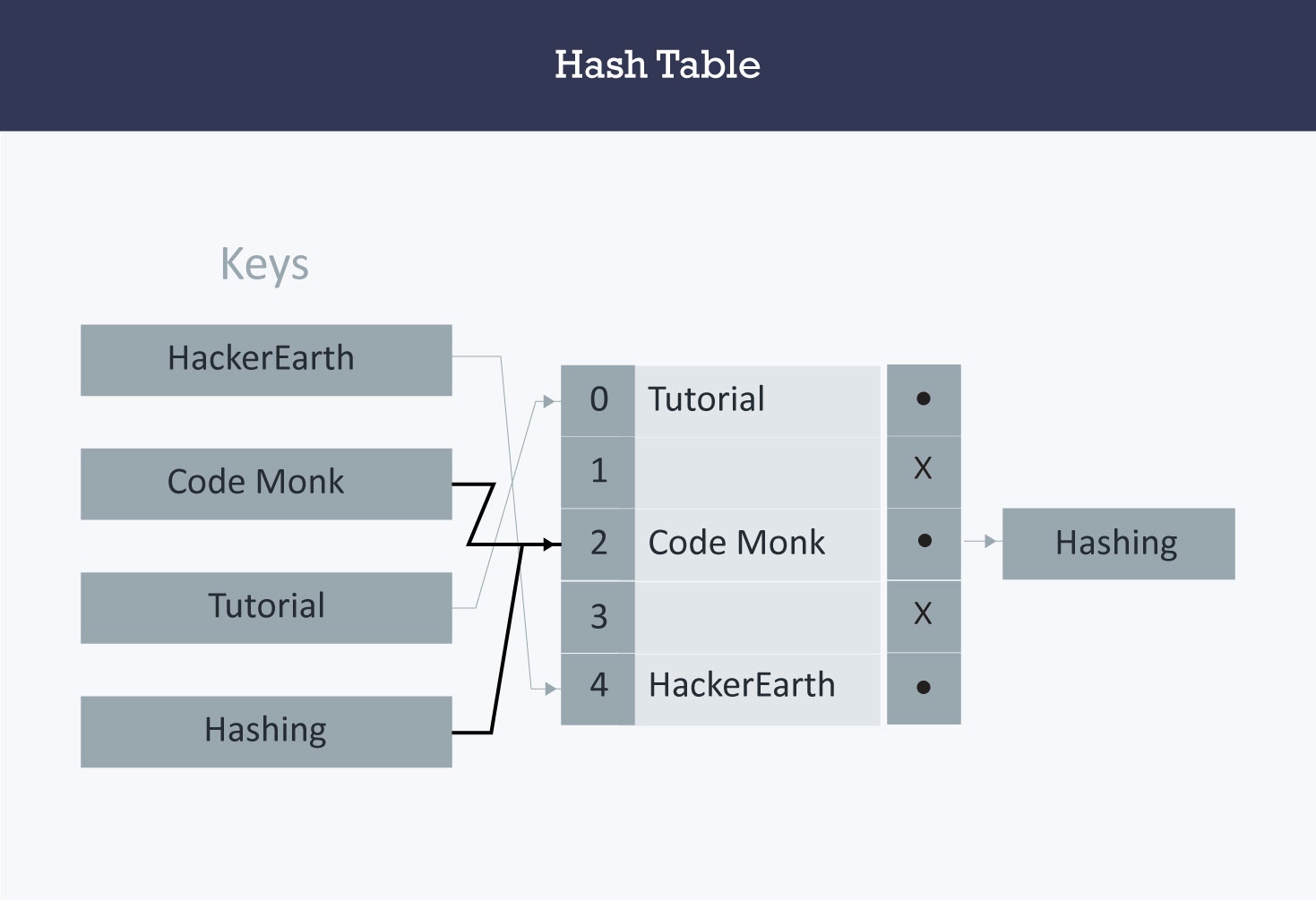Implementation of hash tables with separate chaining (open hashing)

Assumption

Hash function will return an integer from 0 to 19.

vector <string> hashTable;
int hashTableSize=20;


Insert

   void insert(string s)
{
// Compute the index using Hash Function
int index = hashFunc(s);
// Insert the element in the linked list at the particular index
hashTable[index].push_back(s);
}


Search

   void search(string s)
{
//Compute the index by using the hash function
int index = hashFunc(s);
//Search the linked list at that specific index
for(int i = 0;i < hashTable[index].size();i++)
{
if(hashTable[index][i] == s)
{
cout << s << " is found!" << endl;
return;
}
}
}


Linear probing (open addressing or closed hashing)

In open addressing, instead of in linked lists, all entry records are stored in the array itself. When a new entry has to be inserted, the hash index of the hashed value is computed and then the array is examined (starting with the hashed index). If the slot at the hashed index is unoccupied, then the entry record is inserted in slot at the hashed index else it proceeds in some probe sequence until it finds an unoccupied slot.

The probe sequence is the sequence that is followed while traversing through entries. In different probe sequences, you can have different intervals between successive entry slots or probes.

When searching for an entry, the array is scanned in the same sequence until either the target element is found or an unused slot is found. This indicates that there is no such key in the table. The name "open addressing" refers to the fact that the location or address of the item is not determined by its hash value.

Linear probing is when the interval between successive probes is fixed (usually to 1). Let’s assume that the hashed index for a particular entry is index. The probing sequence for linear probing will be:

index = index % hashTableSize
index = (index + 1) % hashTableSize
index = (index + 2) % hashTableSize
index = (index + 3) % hashTableSize

and so on…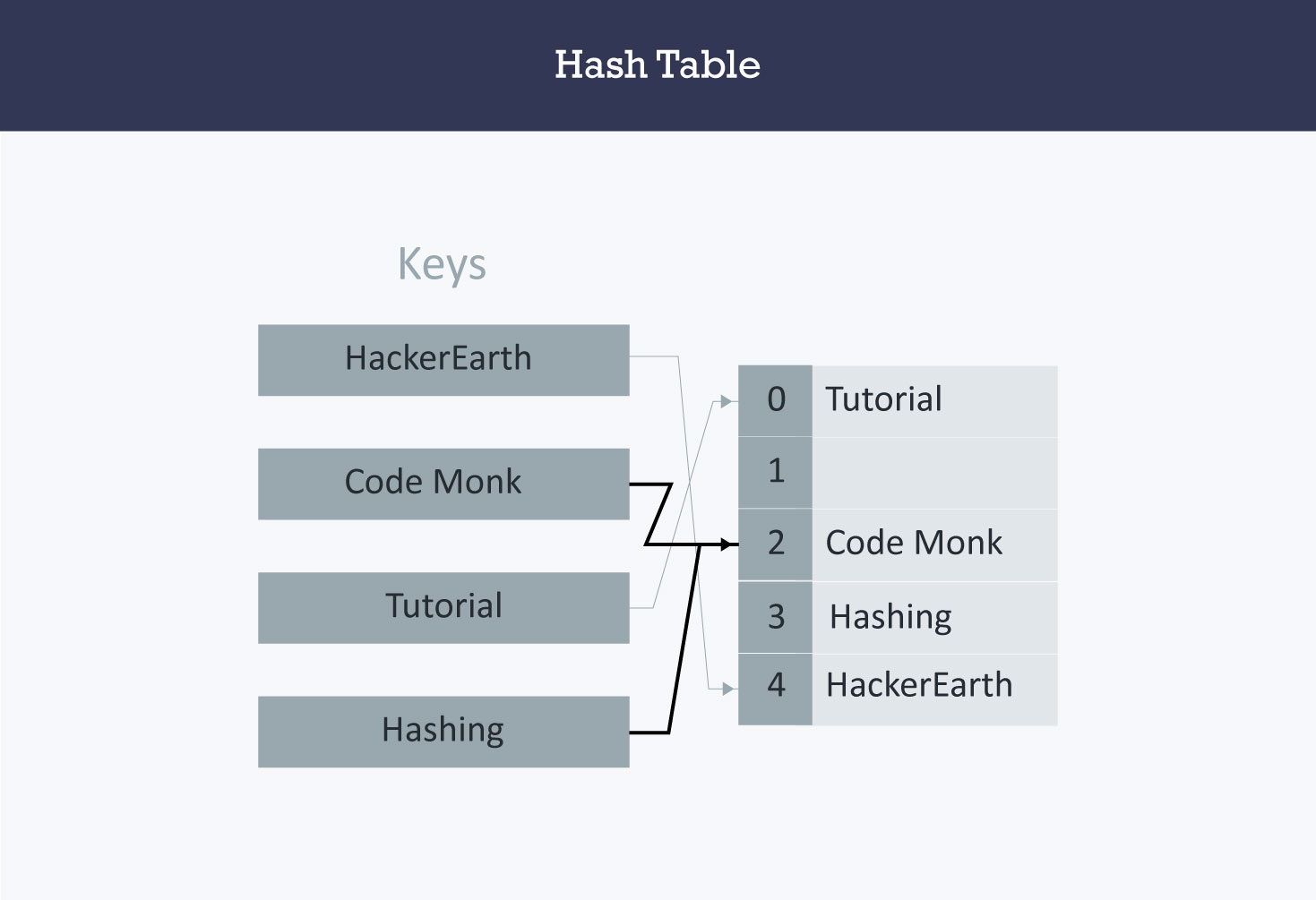Hash collision is resolved by open addressing with linear probing. Since CodeMonk and Hashing are hashed to the same index i.e. 2, store Hashing at 3 as the interval between successive probes is 1.

Implementation of hash table with linear probing

Assumption

• There are no more than 20 elements in the data set.
• Hash function will return an integer from 0 to 19.
• Data set must have unique elements.
 string hashTable;
int hashTableSize = 21;


Insert

void insert(string s)
{
//Compute the index using the hash function
int index = hashFunc(s);
//Search for an unused slot and if the index will exceed the hashTableSize then roll back
while(hashTable[index] != "")
index = (index + 1) % hashTableSize;
hashTable[index] = s;
}


Search

   void search(string s)
{
//Compute the index using the hash function
int index = hashFunc(s);
//Search for an unused slot and if the index will exceed the hashTableSize then roll back
while(hashTable[index] != s and hashTable[index] != "")
index = (index + 1) % hashTableSize;
//Check if the element is present in the hash table
if(hashTable[index] == s)
cout << s << " is found!" << endl;
else
}


Quadratic probing is similar to linear probing and the only difference is the interval between successive probes or entry slots. Here, when the slot at a hashed index for an entry record is already occupied, you must start traversing until you find an unoccupied slot. The interval between slots is computed by adding the successive value of an arbitrary polynomial in the original hashed index.

Let us assume that the hashed index for an entry is index and at index there is an occupied slot. The probe sequence will be as follows:

index = index % hashTableSize
index = (index + 12) % hashTableSize
index = (index + 22) % hashTableSize
index = (index + 32) % hashTableSize

and so on…

Implementation of hash table with quadratic probing

Assumption

• There are no more than 20 elements in the data set.
• Hash function will return an integer from 0 to 19.
• Data set must have unique elements.
string hashTable;
int hashTableSize = 21;


Insert

   void insert(string s)
{
//Compute the index using the hash function
int index = hashFunc(s);
//Search for an unused slot and if the index will exceed the hashTableSize roll back
int h = 1;
while(hashTable[index] != "")
{
index = (index + h*h) % hashTableSize;
h++;
}
hashTable[index] = s;
}


Search

void search(string s)
{
//Compute the index using the Hash Function
int index = hashFunc(s);
//Search for an unused slot and if the index will exceed the hashTableSize roll back
int h = 1;
while(hashTable[index] != s and hashTable[index] != "")
{
index = (index + h*h) % hashTableSize;
h++;
}
//Is the element present in the hash table
if(hashTable[index] == s)
cout << s << " is found!" << endl;
else
}


Double hashing

Double hashing is similar to linear probing and the only difference is the interval between successive probes. Here, the interval between probes is computed by using two hash functions.

Let us say that the hashed index for an entry record is an index that is computed by one hashing function and the slot at that index is already occupied. You must start traversing in a specific probing sequence to look for an unoccupied slot. The probing sequence will be:

index = (index + 1 * indexH) % hashTableSize;
index = (index + 2 * indexH) % hashTableSize;

and so on…

Here, indexH is the hash value that is computed by another hash function.

Implementation of hash table with double hashing

Assumption

• There are no more than 20 elements in the data set.
• Hash functions will return an integer from 0 to 19.
• Data set must have unique elements.
    string hashTable;
int hashTableSize = 21;


Insert

  void insert(string s)
{
//Compute the index using the hash function1
int index = hashFunc1(s);
int indexH = hashFunc2(s);
//Search for an unused slot and if the index exceeds the hashTableSize roll back
while(hashTable[index] != "")
index = (index + indexH) % hashTableSize;
hashTable[index] = s;
}


Search

  void search(string s)
{
//Compute the index using the hash function
int index = hashFunc1(s);
int indexH = hashFunc2(s);
//Search for an unused slot and if the index exceeds the hashTableSize roll back
while(hashTable[index] != s and hashTable[index] != "")
index = (index + indexH) % hashTableSize;
//Is the element present in the hash table
if(hashTable[index] == s)
cout << s << " is found!" << endl;
else
}


Applications

• Associative arrays: Hash tables are commonly used to implement many types of in-memory tables. They are used to implement associative arrays (arrays whose indices are arbitrary strings or other complicated objects).
• Database indexing: Hash tables may also be used as disk-based data structures and database indices (such as in dbm).
• Caches: Hash tables can be used to implement caches i.e. auxiliary data tables that are used to speed up the access to data, which is primarily stored in slower media.
• Object representation: Several dynamic languages, such as Perl, Python, JavaScript, and Ruby use hash tables to implement objects.
• Hash Functions are used in various algorithms to make their computing faster
Contributed by: Prateek Garg